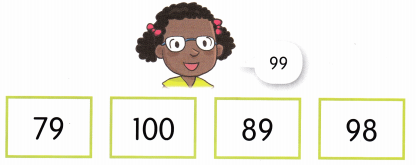# Math in Focus Kindergarten Chapter 8 Answer Key Numbers to 100

Go through the Math in Focus Grade K Workbook Answer Key Chapter 8 Numbers to 100 to finish your assignments.

## Math in Focus Kindergarten Chapter 8 Answer Key Numbers to 100

Lesson 1 Counting by 2s

Count and Write.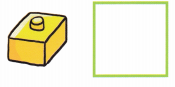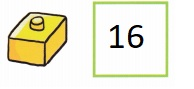Explanation:
There are 16 blocks, Count is 16.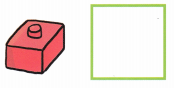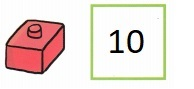Explanation:
There are 10 blocks, Count is 10.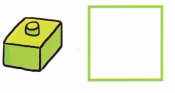Explanation:
There are 7 blocks, Count is 7.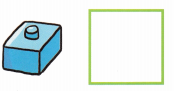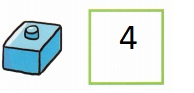Explanation:
There are 4 blocks, Count is 4.

Lesson 2 Counting by 5s

Circle the groups of 5 ants.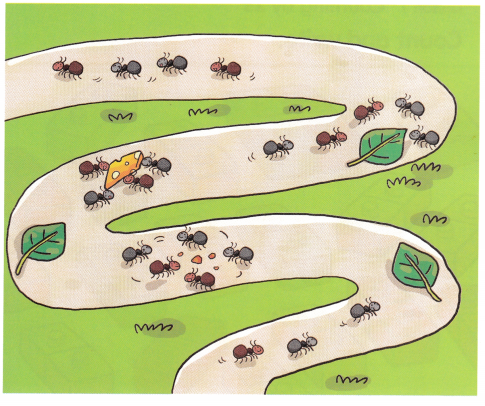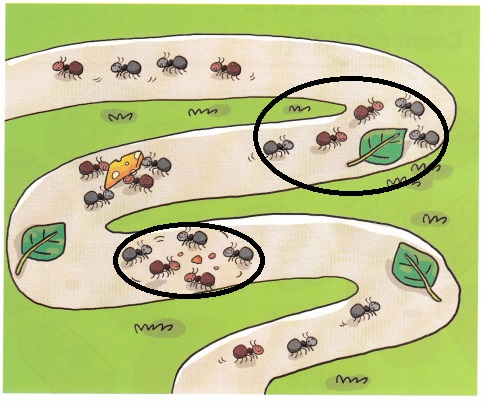Explanation :
Circled the two(2) groups of 5 ants above.

Make the tally.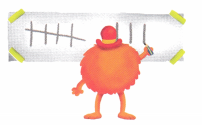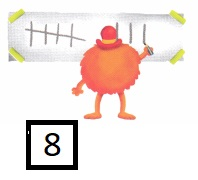Explanation:
Made the tally and result is 8.

Lesson 3 Counting by 10s to 100

How many? Count and circle.

Question 1.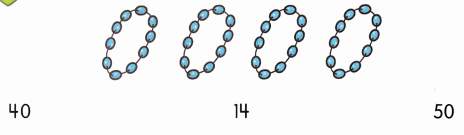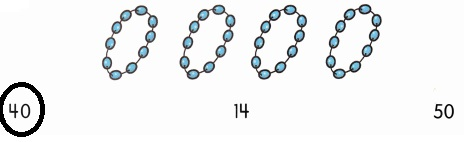Explanation:
Counted and Circled 40.

Question 2.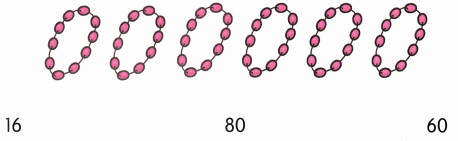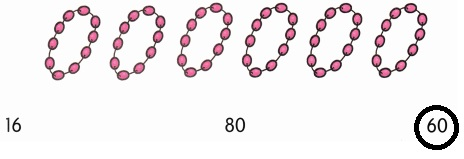Explanation:
Counted and Circled 60.

Question 3.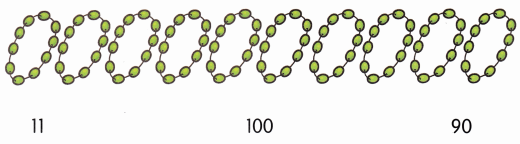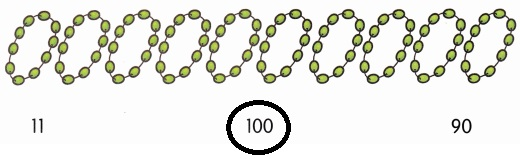Explanation:
Counted and Circled 100.

Circle groups of 10. Then, count and circle.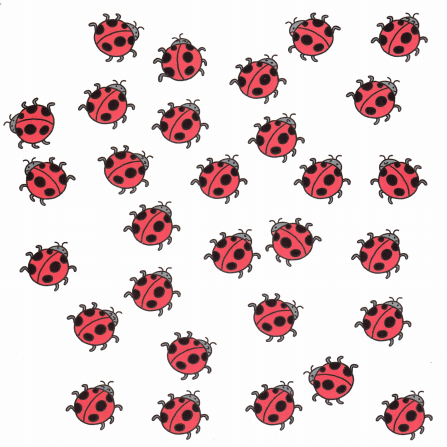How many?
20
30
40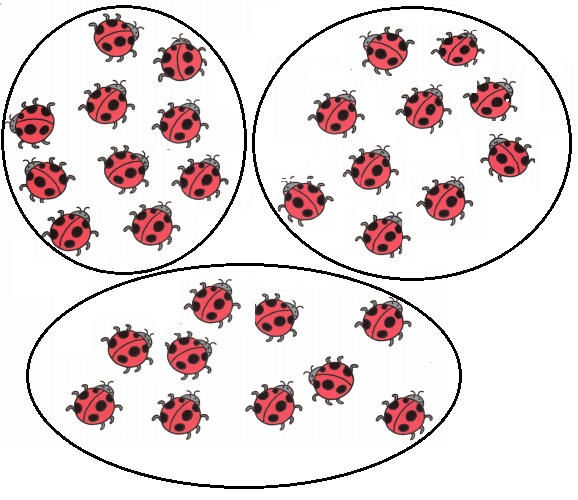Count is 30,

Explanation:
Circled groups of 10. Then counted and circle 30.

Lesson 4 Numbers 20 to 49

Question 1.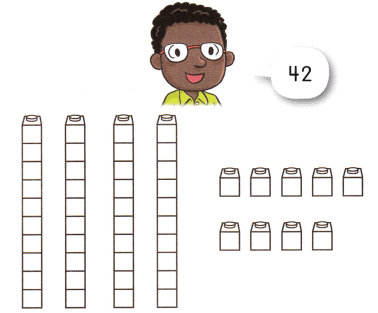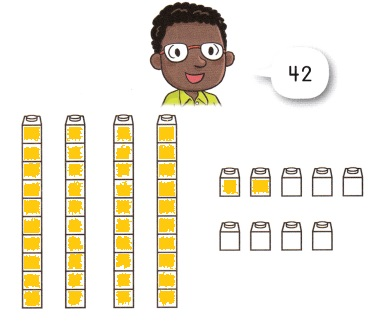Explanation:

Question 2.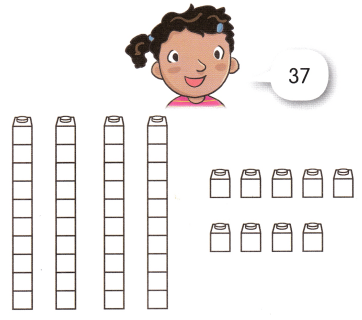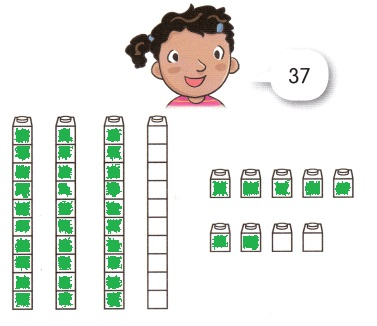Explanation:

How many? Count and Circle.

Question 1.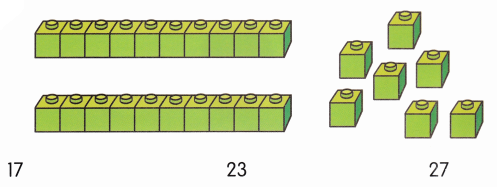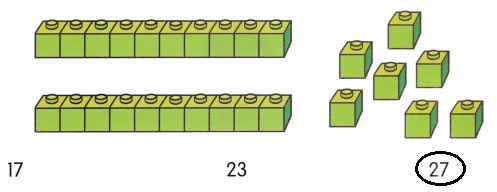Explanation:
Counted 27 and Circled 27.

Question 2.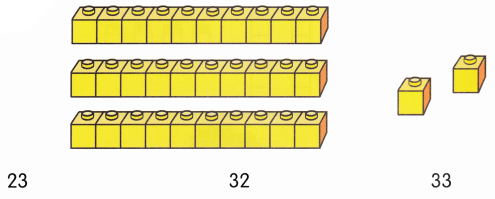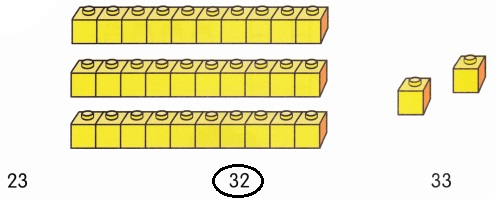Explanation:
Counted 32 and Circled 32.

Question 3.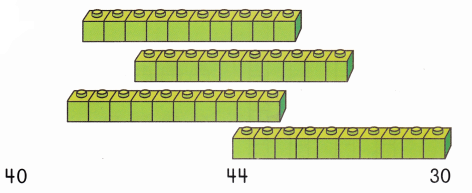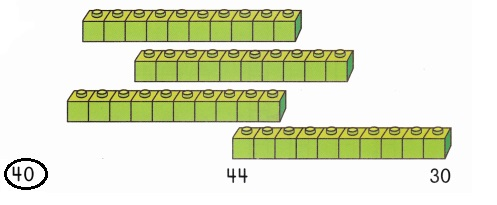Explanation:
Counted 40 and Circled 40.

Complete the sequence. Circle the missing number.

Question 1.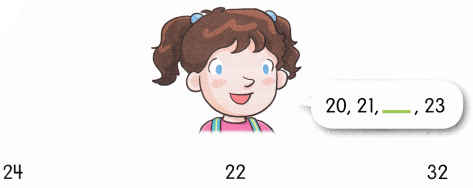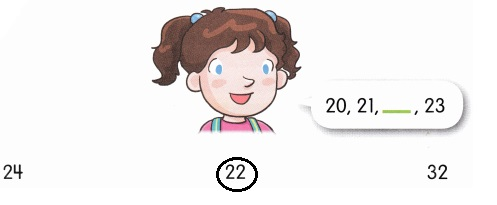Explanation:
Completed the sequence 20,21,22,23.
Circled the missing number 22.

Question 2.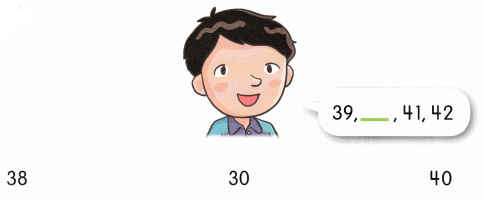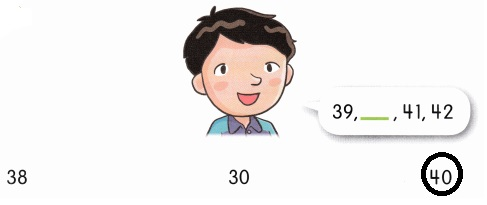Explanation:
Completed the sequence 39,40,41,42.
Circled the missing number 40.

Question 3.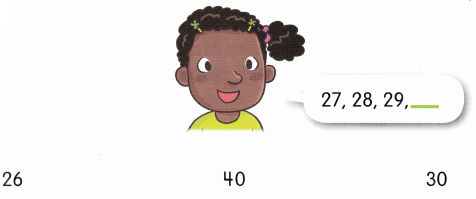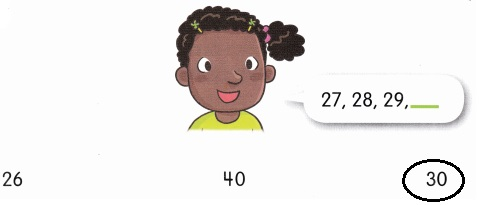Explanation:
Completed the sequence 27,28,29,30.
Circled the missing number 30.

Lesson 5 Numbers 50 to 79

How many? Count and circle.

Question 1.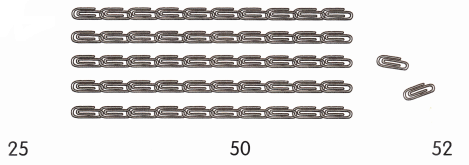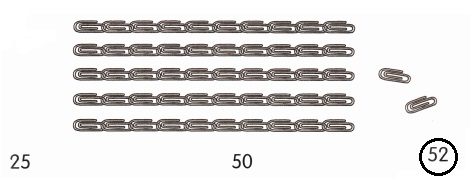Explanation:
Counted and circled 52.

Question 2.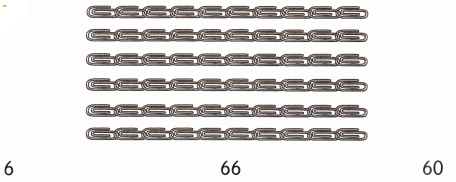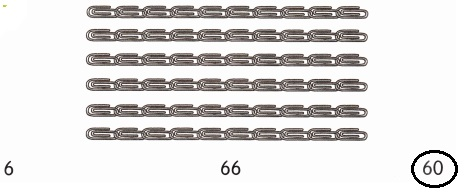Explanation:
Counted and circled 60.

Question 3.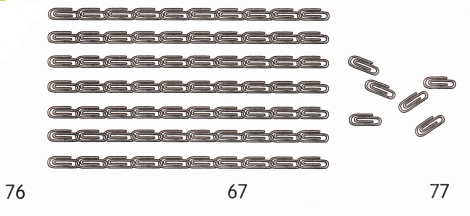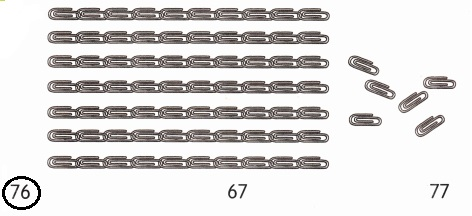Explanation:
Counted and circled 76.

Which is the missing number? Color the balloon.

Question 1.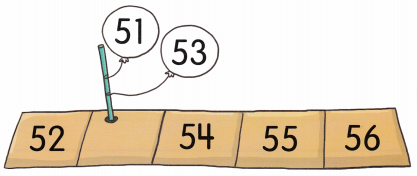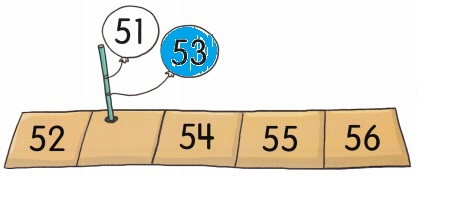Explanation:
Missing number is 53, Colored the balloon with blue.

Question 2.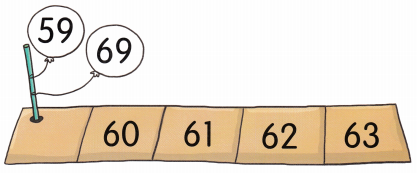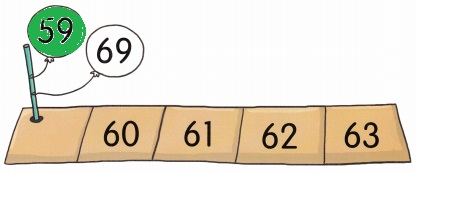Explanation:
Missing number is 59, Colored the balloon with green.

Question 3.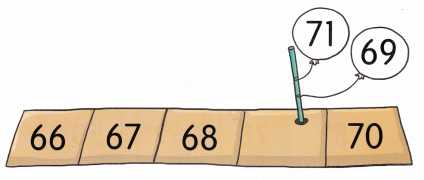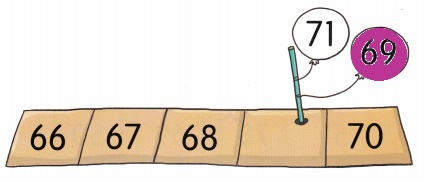Explanation:
Missing number is 69, Colored the balloon with purple.

Color the least number orange. Color the greatest number blue.

Question 1.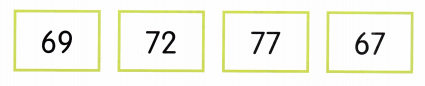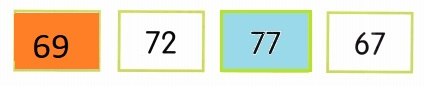Explanation:
Colored the least number 69 with orange.
Colored the greatest number 77 with blue.

Question 2.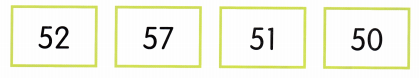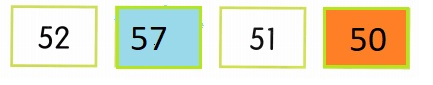Explanation:
Colored the least number 50 with orange.
Colored the greatest number 57 with blue.

Question 3.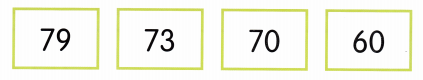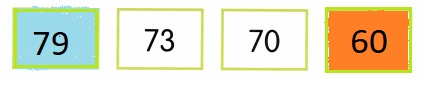Explanation:
Colored the least number 60 with orange.
Colored the greatest number 79 with blue.

Question 4.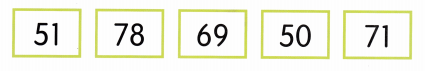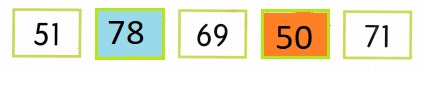Explanation:
Colored the least number 50 with orange.
Colored the greatest number 78 with blue.

Lesson 6 Numbers 80 to 100

How many? Count and circle.

Question 1.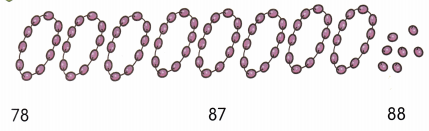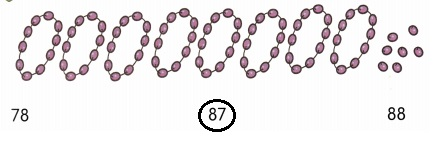Explanation:
Counted 87 and circled 87.

Question 2.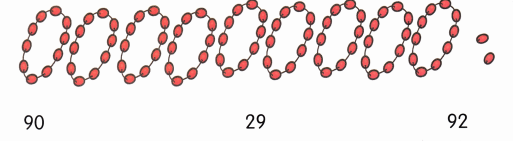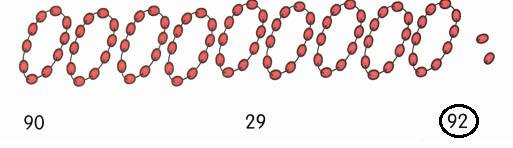Explanation:
Counted 92 and circled 92.

Question 3.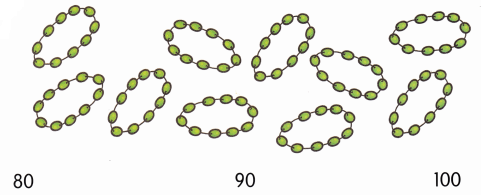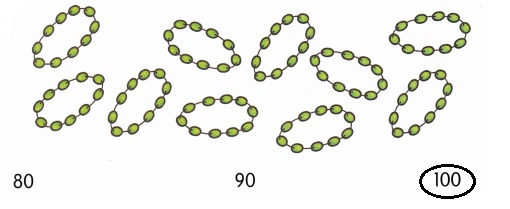Explanation:
Counted 100 and circled 100.

Question 1.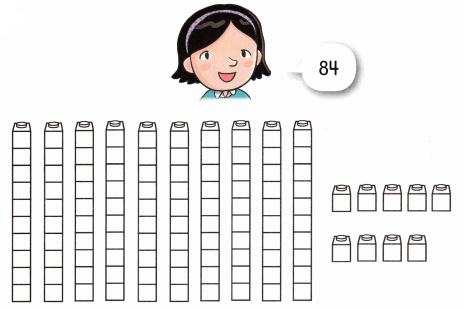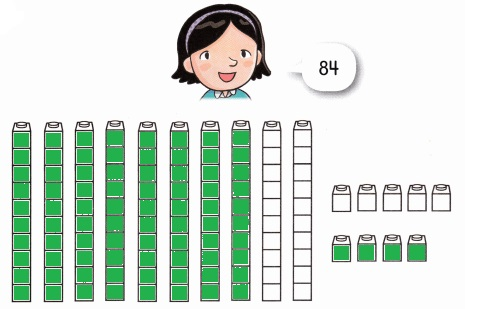Explanation:
Read 84 and colored 84 cubes.

Question 2.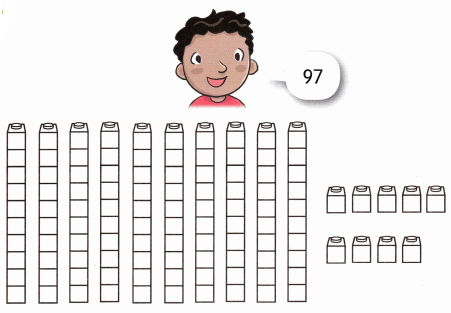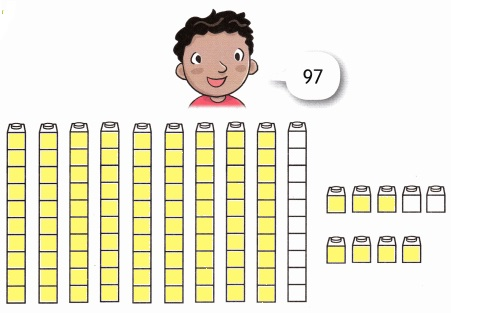Explanation:
Read 97 and colored 97 cubes.

Which is the missing number? Color the flag.

Question 1.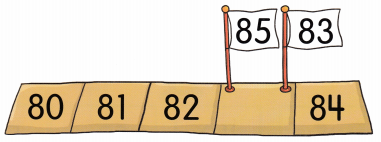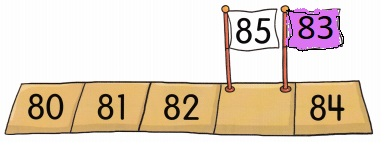Explanation:
The missing numberis 83, Colored the flag 83 with purple.

Question 2.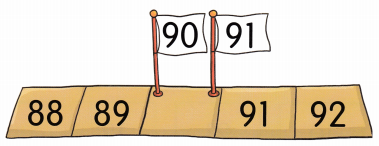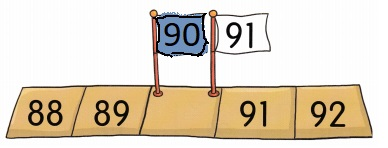Explanation:
The missing number is 90, Colored the flag 90 with blue.

Question 3.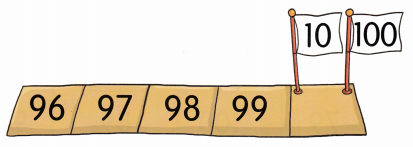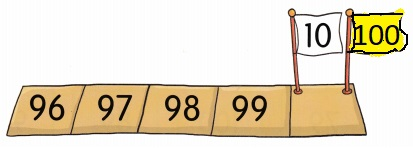Explanation:
The missing number is 100, Colored the flag with Yellow.

Lesson 7 Numbers 1 to 100

What comes before? Color blue. What comes after? Color red.

Question 1.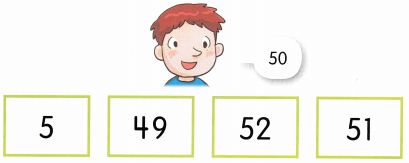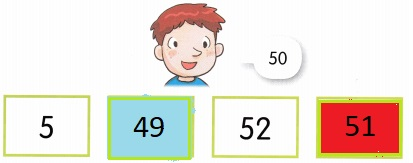Explanation:
Colored the before 50 number 49 with blue.
Colored the after 50 number 51 with red.

Question 2.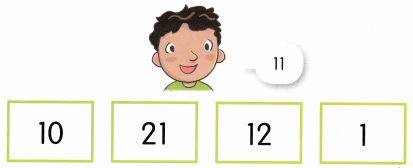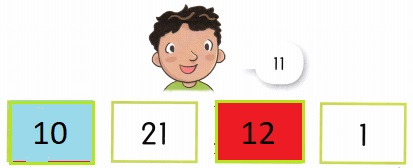Explanation:
Colored the before 11 number 10 with blue.
Colored the after 11 number 12 with red.

Question 3.